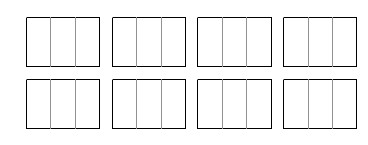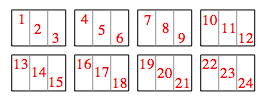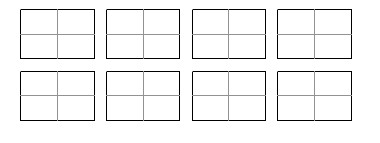### Home > MC2 > Chapter 8 > Lesson 8.2.2 > Problem8-44

8-44.

Study the following division problems and the diagrams that represent them. Answer the question below each diagram.

1. $8 \div \frac { 1 } { 3 }$How many thirds?

$8$ wholes were each divided into $3$ sections. Now count the number of sections to get your answer.There are $24$ thirds.

1. $8 \div \frac { 1 } { 4 }$How many fourths?

See part (a).